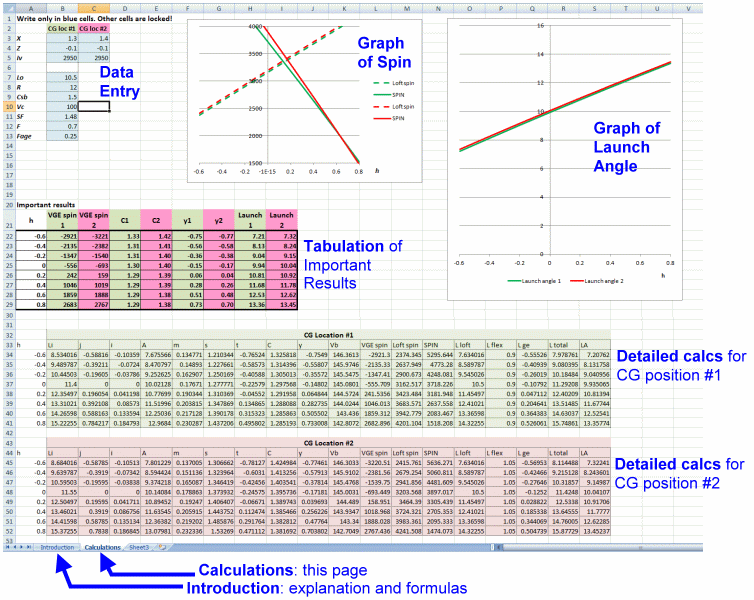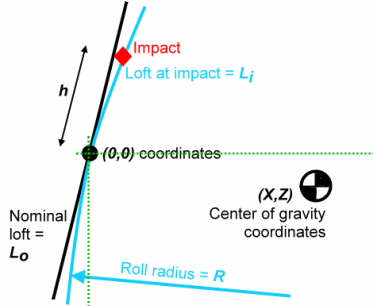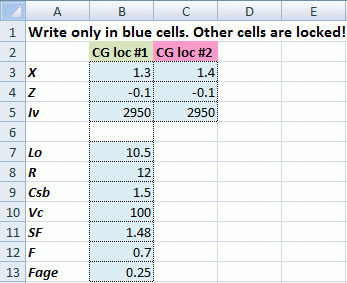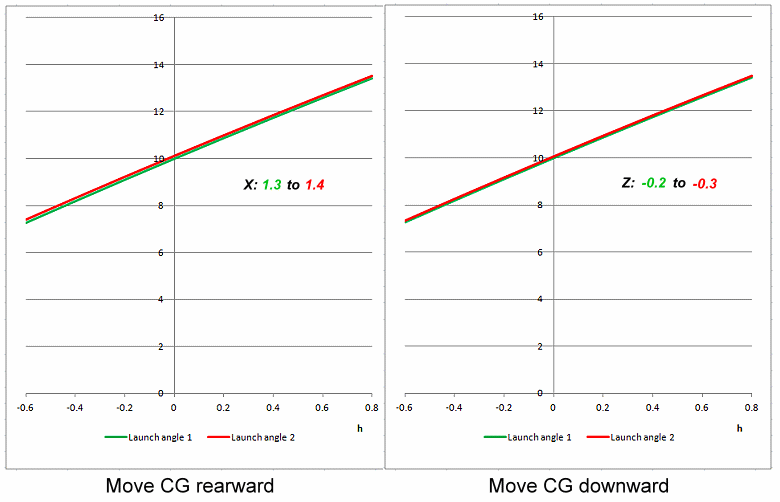for Best Spin and Launch Angle

Dave Tutelman -- August 16, 2013

We have seen the reasons for the CG placement to affect spin and launch angle. Now for some numbers. Let's look at a few cases, and see what moving around the center of gravity does to spin and launch angle.

I built a spreadsheet to do the calculations. You can download a copy for yourself if you want to play with cases and get a better feel for what is going on. Let's cover that first, before we review what playing with the numbers teaches us.

I have made an Excel spreadsheet that will plot the spin and launch angle for two different placements of the center of gravity. You can set up the two placements; the reason for two is to be able to compare and note the effects. Here is an overview screenshot of the calculation sheet, in case you decide to download and use it:The screenshot shows the Calculations page. There is also an Introduction page, which reviews the meaning of each parameter, and gives the formulas used in the calculations.

At the upper left is the input area. You can only enter data in the light blue cells of the input area. The rest of the spreadsheet is protected from inadvertent entries, so you don't accidentally corrupt the formulas.

You can input the data for two different placements of the center of gravity. The calculations, results, and graphs are color-coded, green for placement #1 and red for placement #2.The spreadsheet is based on coordinates shown in the accompanying diagram. We require some coordinate system, because first and foremost we need to describe the position of the CG; that is the primary focus of our study.

The origin (the [0,0] point) of our coordinates is the center of the clubface. Specifically, it is the face at exactly the point where the loft is the nominal loft of the clubhead. If we are studying a 10.5º driver, then [0,0] occurs where the face is slanted 10.5º from vertical. In the diagram the X and Z axes are green dotted lines.

The center of gravity is therefore specified with X being the horizontal distance behind the midpoint of the clubface, and Z being the vertical distance above the midpoint of the clubface. The example in the diagram shows the CG about 1.3" behind the clubface and 0.2" below the axis. So the coordinates of the CG would be X=1.3 and Z=-0.2, or [1.3, -0.2].

All input is done in the blue cells at the upper left of the worksheet, shown in the screenshot below. The input parameters are:X, Z The coordinates of the CG, in inches. There are two columns here, one for each of the CG locations. Iv The vertical moment of inertia of the clubhead. Modern drivers have this value close to 2950 gram-cm-squared, so that is the default in the spreadsheet. Why two columns? Because some methods that clubhead designers use to move the CG may also change the MOI, so the two CG locations may need different values for MOI. Lo The nominal loft of the clubhead, in degrees. R The face roll, specified as the radius of curvature in inches. The default of 12" is around the middle of the range for modern driver heads. Csb Coefficient of shaft bend, in degrees per inch. This is a simple number that Jeff Summitt tells me is a pretty good approximation. It will change with clubhead speed and shaft flex profile, but probably not by a factor as much as two. Vc Clubhead speed at impact, in MPH. SF Smash factor. The default is the theoretical maximum for a 200g driver head. F The horizontal distance from center-face to the shaft centerline. In coordinate terms, it is the X-coordinate of the shaft centerline. The default of 0.7" is a typical value for a modern driver. It might be lower for, say, an offset driver or a fairway wood. Fage The fraction of the angle of loft change during impact, due to gear effect, that gives us the effective loft for computing launch angle. The analysis says it should be 1/4, which is the default value. (That is, 1/4 of the angle change during impact is the effective angle for computing the change of launch angle.) But you can tweak it to a higher number if you want to test the assumptions I made in the analysis.

Results

Let's look at some graphs from the spreadsheet, and see what they tell us about how we affect spin, launch angle, and ultimately distance by moving the clubhead's center of gravity.

SpinHere are spin graphs for two runs of the spreadsheet. One moves the center of gravity rearward in the clubhead by 0.1", the other downward by 0.1". Except for the specific CG locations in the diagram, the spreadsheet was primed with the default values shown in the previous section.

Before we talk about the results, a few words about the graphs themselves.  Each graph has two sets of green curves (CG position #1) and two sets of red curves (CG position #2). The solid curve is the total spin, and definitely the most interesting. But there is also something to be learned from the dashed curve, that I call "loft spin" for short. The dashed curve is the spin created by the loft of the club as it comes to impact, including both the loft built into the clubhead and the added loft due to shaft bend. The solid curve is the dashed curve minus the gear effect topspin.

Now for the results themselves...

Moving the CG rearward has a mixed value, but most of it has the effect of increasing the backspin. This is counter to the older conventional wisdom, which holds that a readward CG reduces backspin. The higher on the clubface you hit the ball the less the spin penalty, but the curves don't cross until more than a half inch above mid-face. At mid-face, the rearward CG has about 160rpm more backspin. That is significant, though far from huge.

Of course, we know that a mid-face strike is not where you want to hit the ball to maximize distance. Suppose we try 0.4" above the center. Looking at the curves, we can see that the spin difference between the green and red curves has almost disappeared. It is only 65rpm.

 X = 1.3 X = 1.4 Ball Speed Spin LA Carry Dist Ball Speed Spin LA Carry Dist h = 0 145 3642 9.9 230 145 3801 10.0 229 h = .4 144 2797 11.6 232 143 2862 11.8 230
Let's see how that translates into distance. For the table at the right, we plug the computed launch parameters into TrajectoWare Drive and find the carry distance. In each case the rearward CG results in less distance, but only by a yard or two.

The bottom line is that moving the CG rearward makes small, almost unmeasurable differences in distance -- and the difference might go either way.

 Z = -0.1 Z = -0.2 Ball Speed Spin LA Carry Dist Ball Speed Spin LA Carry Dist h = 0 145 3642 9.9 230 145 3274 10.0 231 h = .4 144 2797 11.6 231 144 2415 11.7 229
Moving the CG downward, on the other hand, has a pretty large payoff in terms of spin. We knew this just from the visual description on the previous page, but let's quantify it now. The total spin lines for positions 1 and 2 are almost parallel and are 370-400rpm apart. Of course, the lower CG enhances favorable gear effect and gives lower spin. Let's see how this measures up in terms of distance, for a center-face strike and a high-face (h=0.4") strike.

The results shown in the table are very surprising. Despite the lower spin for the low CG position, it seems to lose distance. Not a lot of distance -- only 1or 2 yards -- but distance that we thought we would gain instead of lose. We will discuss the reason for this surprising result on the next page. It involves the shape of the "launch space", the plot of distance vs launch angle and spin. The conventional wisdom says you always want lower spin and higher launch angle, but we have demonstrated here that is not always the case.

Launch angleThere is a lot less to be said about the variation of launch angle with CG motion -- and that in itself is a surprise.
• Fore and aft movement of the CG changes the shaft bend by .15º for every .1" of CG movement. That will change the launch angle, but by less than .15º. It appears to be roughly .1º of launch angle change for every .1" of CG movement. Barely measurable, it's just one place in the tenths column.
• Up and down movement of the CG doesn't change shaft bend at all. The only way it can change launch angle is through head rotation during impact. That is not a very efficient way to affect launch angle; a .1" CG motion gives less than a .1º change of launch angle.
Both these are much less than I had expected. Perhaps clubhead rotation works by a different mechanism than simply changing the loft during impact. In fact, I postulated a completely different mechanism and tried some back-of-the-envelope calculations. I got almost the same result, so perhaps it is true. Unfortunately, I don't have any good experimental data relating CG placement to launch angle; therefore, I don't have any yardstick to measure whether my launch angle analysis corresponds to reality.

On the next page, we summarize, discuss, and try to explain these results. This leads to a discussion of how to design and fit a driver for a given golfer.

Last updated - Mar 11, 2014